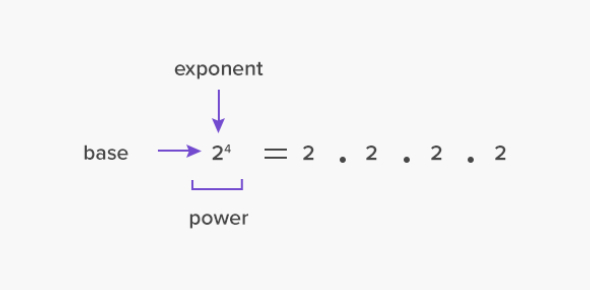# Real Numbers: Numerical Word Problems Quiz

10 Questions | Attempts: 5892
ShareSettingsThis quiz is about Real Numbers, and you will be asked some questions on numerical word problems. There are different ways in which math problems can be described, and using some wordplay, some people can find an obvious math problem to be too hard to solve. Do you need some practice on numerical word problems? If so, then the quiz below is for you and is perfect to help you iron out your skills. Give it a shot!

• 1.
In how many years will a sum of Rs. 800 at 10% per annum compound interest, compounded semiannually becomes Rs. 926.10 ?
• A.

1*1/2

• B.

1*2/3

• C.

2*1/3

• D.

2*1/2

• 2.
In a 100m race, Kamal defeats Bimal by 5 seconds. If the speed of Kamal is 18 Kmph, then the speed of Bimal is
• A.

15.4 kmph

• B.

14.5 kmph

• C.

14.4 kmph

• D.

14 kmph

• 3.
A train, 240 in long crosses a man ‘walking along the line in opposite direction at the rat4 of 3 kmph in 10 seconds. The speed of the train is
• A.

63 kmph

• B.

75 kmph

• C.

83.4 kmph

• D.

86.4 kmph

• 4.
A boatman rows 1 km in 5 minutes, along the stream and 6 km in I hour against the stream. The speed of the stream is
• A.

3 kmph

• B.

6 kmph

• C.

10 kmph

• D.

12 kmph

• 5.
A can complete 1/3 of a work in 5 days and B, 2/5of the work in 10 days. In how many days both A and B together can complete the work ?
• A.

10

• B.

7*3/8

• C.

8*4/5

• D.

7*1/2

• 6.
7 men can complete a piece of work in 12 days. How many additional men will be required to complete double the work in 8 days ?
• A.

28

• B.

21

• C.

14

• D.

7

• 7.
One pipe fills a water tank three times faster than another pipe. If the two pipes together can fill the empty tank in 36 minutes, then how much time will the slower pipe alone take to fill the tank ?
• A.

1 hour 21 minutes

• B.

1 hour 48 minutes

• C.

2 hours

• D.

2 hour 24 minutes

• 8.
In an examination, a student scores 4 marks for every correct answer and loses 1 mark for every wrong answer. A student attempted tempted all the 200 questions and scored, in all 200 marks.The number of questions, he answered correctly was
• A.

82

• B.

80

• C.

68

• D.

60

• 9.
The average of odd numbers up to 100 is
• A.

50.5

• B.

50

• C.

49.5

• D.

49

• 10.
If A’s income is 25% less than B’s income, by how much per cent is B’s income more than that of A ?
• A.

25

• B.

30

• C.

33*1/3

• D.

66*2/3Back to top
×

Wait!
Here's an interesting quiz for you.